# Selina Concise Physics Class 10 ICSE Solutions Calorimetry

## Selina Concise Physics Class 10 ICSE Solutions Calorimetry

APlusTopper.com provides step by step solutions for Selina Concise ICSE Solutions for Class 10 Physics Chapter 11 Calorimetry. You can download the Selina Concise Physics ICSE Solutions for Class 10 with Free PDF download option. Selina Publishers Concise Physics for Class 10 ICSE Solutions all questions are solved and explained by expert teachers as per ICSE board guidelines.

Selina ICSE Solutions for Class 10 Physics Chapter 11 Calorimetry

Exercise 11(A)

Solution 1.

The kinetic energy due to random motion of the molecules of a substance is known as its heat energy.

Solution 2.

S.I. unit of heat is joule (symbol J).

Solution 3.

One calorie of heat is the heat energy required to raise the temperature of 1 g of water from 14.5oC to 15.5 oC.
1 calorie = 4.186 J

Solution 4.

One kilo-calorie of heat is the heat energy required to raise the temperature of 1 kg of water from 14.5oC to 15.5oC.

Solution 5.

The quantity which determines the direction of flow of heat between two bodies kept in contact is called temperature.
S.I. unit kelvin (K).

Solution 6.

 Heat Temperature The kinetic energy due to random motion of the molecules of a substance is known as its heat energy. The quantity which determines the direction of flow of heat between two bodies kept in contact is called temperature. S.I. unit joule (J). S.I. unit kelvin (K). It is measured by the principle of calorimetry. It is measured by a thermometer.

Solution 7.

The measurement of the quantity of heat is called calorimetry.

Solution 8.

The heat capacity of a body is the amount of heat energy required to raise its temperature by 1oC or 1K.
S.I. unit is joule per kelvin (JK-1).

Solution 9.

The specific heat capacity of a substance is the amount of heat energy required to raise the temperature of unit mass of that substance through by 1oC (or 1K).
S.I. unit is joule per kilogram per kelvin (Jkg-1K-1).

Solution 10.

Heat capacity = Mass x specific heat capacity

Solution 11.

• Heat capacity of the body is the amount of heat required to raise the temperature of (whole) body by 1oC whereas specific heat capacity is the amount of heat required to raise the temperature of unit mass of the body by 1oC.
• Heat capacity of a substance depends upon the material and mass of the body. Specific heat capacity of a substance does not depend on the mass of the body.
• S.I. unit of heat capacity is JK-1 and S.I. unit of specific heat capacity is Jkg-1K-1.

Solution 12.

Water has the highest specific heat capacity.

Solution 13.

Specific heat capacity of water=4200 J kg-1 K-1.

Solution 14.

1. The heat capacity of a body is 50JK-1 means to increase the temperature of this body by 1K we have to supply 50 joules of energy.
2. The specific heat capacity of copper is 0.4Jg-1K-1 means to increase the temperature of one gram of copper by 1K we have to supply 0.4 joules of energy.

Solution 16.

The quantity of heat energy absorbed by a body depends on three factors :

1. Mass of the body – The amount of heat energy required is directly proportional to the mass of the substance.
2. Nature of material of the body – The amount of heat energy required depends on the nature on the substance and it is expressed in terms of its specific heat capacity c.
3. Rise in temperature of the body – The amount of heat energy required is directly proportional to the rise in temperature.

Solution 17.

The expression for the heat energy Q
Q = mcΔt (in joule)

Solution 18.

Heat capacity of liquid A is less than that of B.
As the substance with low heat capacity shows greater rise in temperature.

Solution 19.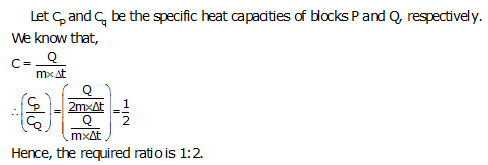Solution 20.

The principle of method of mixture:
Heat energy lost by the hot body = Heat energy gained by the cold body.
This principle is based on law of conservation of energy.

Solution 21.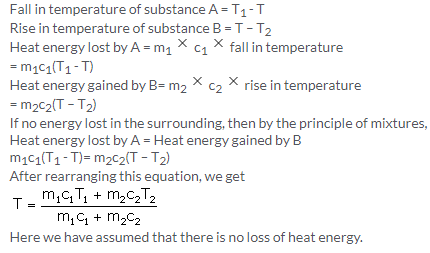Solution 22.

In the absence of water, if on a cold winter night the atmospheric temperature falls below 0oC, the water in the fine capillaries of plant will freeze, so the veins will burst due to the increase in the volume of water on freezing. As a result, plants will die and the crop will be destroyed. In order to save the crop on such cold nights, farmers fill their fields with water because water has high specific heat capacity, so it does not allow the temperature in the surrounding area of plants to fall up to 0oC.

Solution 23.

The specific heat capacity of water is very high. It is about five times as high as that of sand. Hence the heat energy required for the same rise in temperature by a certain mass of water will be nearly five times than that required by the same mass of sand. Similarly, a certain mass of water will give out nearly five times more heat energy than that given by sand of the same mass for the same fall in temperature. As such, sand gets heated or cooled more rapidly as compared to water under the similar conditions. Thus a large difference in temperature is developed between the land and the sea due to which land and sea breezes are formed. These breezes make the climate near the sea shore moderate.

Solution 24.

The reason is that water does not cool quickly due to its large specific heat capacity, so a hot water bottle provides heat energy for fomentation for a long time.

Solution 25.

By allowing water to flow in pipes around the heated parts of a machine, heat energy from such part is removed. Water in pipes extracts more heat from surrounding without much rise in its temperature because of its large specific heat capacity. So, Water is used as an effective coolant.

Solution 26.

2. To avoid freezing of wine and juice bottles.

Solution 28.

A calorimeter is a cylindrical vessel which is used to measure the amount of heat gained or lost by a body when it is mixed with other body.
It is made up of thin copper sheet because:

1. Copper is a good conductor of heat, so the vessel soon acquires the temperature of its contents.
2. Copper has low specific heat capacity so the heat capacity of calorimeter is low and the amount of heat energy taken by the calorimeter from its contents to acquire the temperature of its contents is negligible.

Solution 29.

By making the base of a cooking pan thick, its thermal capacity becomes large and it imparts sufficient heat energy at a low temperature to the food for its proper cooking. Further it keeps the food warm for a long time, after cooking.

Solution 1 (MCQ).

JK-1

Solution 2 (MCQ).

J kg-1K-1

Solution 3 (MCQ).

4200 J kg-1K-1

Numericals

Solution 1.

The size of 1 degree on the Kelvin scale is the same as the size of 1 degree on the Celsius scale. Thus, the difference (or change) in temperature is the same on both the Celsius and Kelvin scales.
Therefore, the corresponding rise in temperature on the Kelvin scale will be 15K.

Solution 2.Solution 3.

1. We know that heat energy needed to raise the temperature by 15o is = heat capacity x change in temperature.
Heat energy required= 966 J K-1 x 15 K = 14490 J.
2. We know that specific heat capacity is = heat capacity/ mass of substance
So specific heat capacity is = 966 / 2=483 J kg-1 K-1.

Solution 4.Solution 5.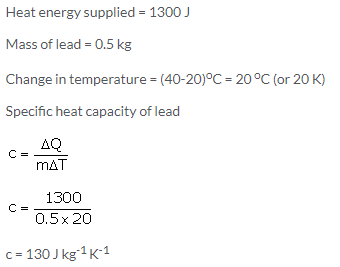Solution 6.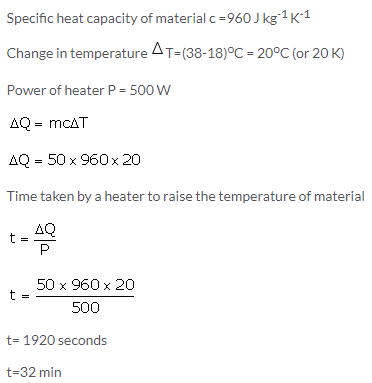Solution 7.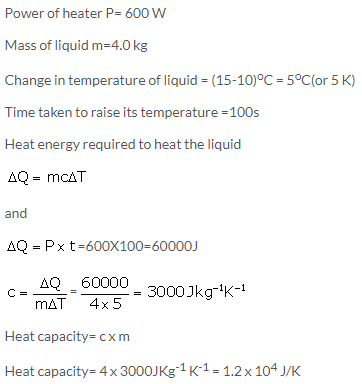Solution 8.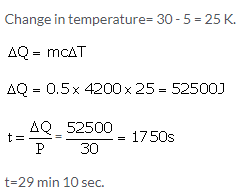Solution 9.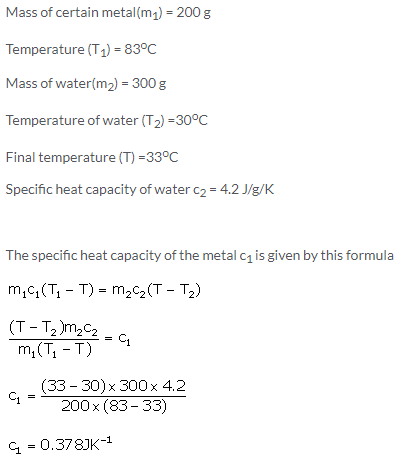Solution 10.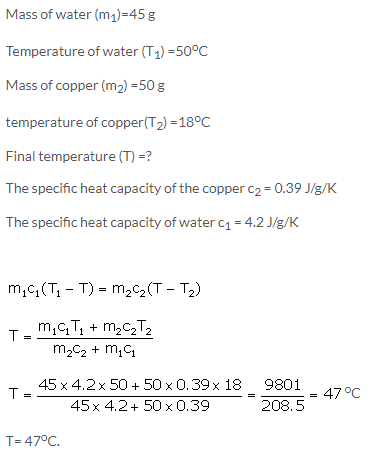Solution 11.Solution 12.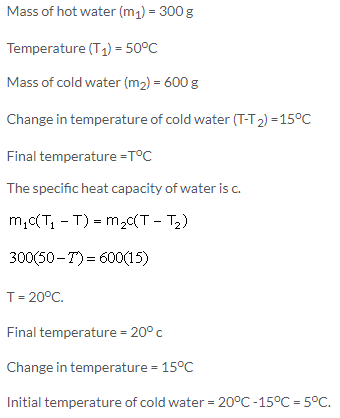Solution 13.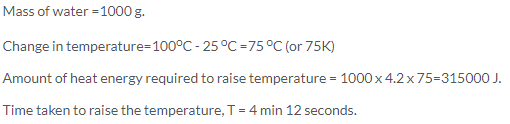Exercise 11(B)

Solution 1.

(a) The process of change from one state to another at a constant temperature is called the change of phase of substance.
(b) There is no change in temperature during the change of phase.
(c) Yes, the substance absorbs or liberates heat during the change of phase.

Solution 2.

Melting: The change from solid to liquid phase on heating at a constant temperature is called melting.
Melting point: The constant temperature at which a solid changes to liquid is called the melting point.

Solution 3.

1. Average kinetic energy of molecules changes.
2. Average potential energy of molecules changes.

Solution 4.

(a) Average kinetic energy does not change.
(b) Average potential energy increases.
Explanation: When a substance is heated at constant temperature (i.e. during its phase change state), the heat supplied makes the vibrating molecules gain potential energy to overcome the intermolecular force of attraction and move about freely. This means that the substance changes its form.

However, this heat does not increase the kinetic energy of the molecules, and hence, no rise in temperature takes place during the change in phase of a substance.
This heat supplied to the substance is known as latent heat and is utilized in changing the state of matter without any rise in temperature.

Solution 5.

The melting point of ice decreases by the presence of impurity in it.
Use: In making the freezing mixture by adding salt to ice. This freezing mixture is used in preparation of ice creams.

Solution 6.

The melting point of ice decreases by the increase in pressure. The melting point of ice decrease by 0.0072oC for every one atmosphere rise in pressure.

Solution 7.

(a) AB part shows rise in temperature of solid from 0 to T1oC, BC part shows melting at temperature T1oC, CD part shows rise in temperature of liquid from T1oC to T3oC , DE part shows the boiling at temperature T3oC.
(b) T1oC.
(c) T3oC.

Solution 8.Solution 9.

Boiling: The change from liquid to gaseous phase on heating at a constant temperature is called boiling.
Boiling point: The particular temperature at which vaporization occurs is called the boiling point of liquid.

Solution 10.

Volume of water wills increases when it boils at 100oC.

Solution 11.

The boiling point of water increases on adding salt.

Solution 12.

The boiling point of a liquid increases on increasing the pressure.

Solution 14.

In a pressure cooker, the water boils at about 120oC to 125oC.

Solution 15.

This is because at high altitudes atmospheric pressure is low; therefore boiling point of water decreases and so it does not provide the required heat energy for cooking.

Solution 16.

(a) When ice melts, its volume decreases.
(b) Decrease in pressure over ice increases its meltingpoint.
(c) Increase in pressure increases the boiling point of water.
(d)A pressure cooker is based on the principle that boiling point of water increases with the increase in pressure.
(e) The boiling point of water is defined as the constant temperature at which water changes to steam.
(f) water can be made to boil at 115°C by increasing pressure over its surface.

Solution 17.

Latent heat: The heat energy exchanged in change of phase is not externally manifested by any rise or fall in temperature, it is considered to be hidden in the substance and is called the latent heat.

Solution 18.

The quantity of heat required to convert unit mass of ice into liquid water at 00C (melting point) is called the specific latent heat of fusion of ice.
Its S.I. unit is Jkg-1.

Solution 19.

Specific latent heat of ice: 336000 J kg-1.

Solution 20.

It means 1 g of ice at 0oC absorbs 360 J of heat energy to convert into water at 0oC.

Solution 21.

Latent heat is absorbed by ice.

Solution 22.

1 g of water at 0oC has more heat than 1 g of ice at 0oC. This is because ice at 0oC absorbs 360 J of heat energy to convert into water at 0oC.

Solution 23.

(a) 1 g ice at 0oC requires more heat because ice would require additional heat energy equal to latent heat of melting.
(b) 1 g ice at 0oC first absorbs 336 J heat to convert into 1 g water at 0oC.

Solution 24.

This is because 1 g of ice at 0oC takes 336 J of heat energy from the mouth to melt at 0oC. Thus mouth loses an additional 336 J of heat energy for 1 g of ice at 0oC than for 1g of water at 0oC. Therefore cooling produced by 1 g of ice at 0oC is more than for 1g of water at 0oC.

Solution 25.

This is because 1 g of ice at 0oC takes 336 J of heat energy from the bottle to melt into water at 0oC. Thus bottle loses an additional 336 J of heat energy for 1 g of ice at 0oC than for 1 g iced water at 0oC. Therefore bottled soft drinks get cooled, more quickly by the ice cubes than by iced water.

Solution 26.

The reason is that after the hail storm, the ice absorbs the heat energy required for melting from the surrounding, so the temperature of the surrounding falls further down and we feel colder.

Solution 27.

The reason is that the heat energy required for melting the frozen lake is absorbed from the surrounding atmosphere. As a result, the temperature of the surrounding falls and it became very cold.

Solution 29.

1. The reason is that the specific latent heat of fusion of ice is sufficiently high, so when the water of lake freezes, a large quantity of heat has to be released and hence the surrounding temperature becomes pleasantly warm.
2. Heat supplied to a substance during its change of state, does not cause any rise in its temperature because this is latent heat of phase change which is required to change the phase only.

Solution 1 (MCQ).

J kg-1

Solution 2 (MCQ).

80cal g-1

Numericals

Solution 1.

Mass of ice=10g = 0.01kg
Amount of heat energy absorbed, Q=5460J
Specific latent heat of fusion of ice=?
Specific heat capacity of water = 4200Jkg-1K-1
Amount of heat energy required by 10g (0.01kg) of water at 0oC to raise its temperature by 50oC= 0.01X4200X50=2100J.
Let Specific latent heat of fusion of ice=L Jg-1.
Then,
Q = mL + mcΔT
5460 J = 10 x L + 2100J
L = 336Jg-1.

Solution 2.

Mass of water m = 5.0 g
specific heat capacity of water c = 4.2 J g-1 K-1
specific latent heat of fusion of iceL =336 J g-1
Amount of heat energy released when 5.0 g of water at 20oC changes into water at 0oC = 5 x 4.2 x 20 = 420 J.
Amount of heat energy released when 5.0g of water at 0oC changes into ice at 0oC=5x336J=1680J.
Total amount of heat released =1680 J + 420 J = 2100 J.

Solution 3.Solution 4.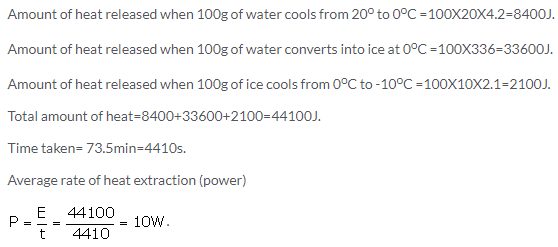Solution 5.

Mass of ice m1 =17 g
Mass of water m2 =40 g.
Change in temperature =34-0=34K
Specific heat capacity of water is 4.2Jg-1K-1.
Assuming there is no loss of heat, heat energy gained by ice (latent heat of ice), Q= heat energy released by water
Q = 40 x 34 x 4.2 = 5712 J.Solution 6.

Let whole of the ice melts and let the final temperature of the mixture be ToC.
Amount of heat energy gained by 10g of ice at -10oC to raise its temperature to 0oC= 10x10x2.1=210J
Amount of heat energy gained by 10g of ice at 0oC to convert into water at 0oC=10×336=3360 J
Amount of heat energy gained by 10g of water (obtained from ice) at 0oC to raise its temperature to ToC = 10×4.2x(T-0)=42T
Amount of heat energy released by 10g of water at 10oC to lower its temperature to ToC = 10×4.2x(10-T)=420-42T
Heat energy gained = Heat energy lost
210 + 3360 + 42T = 420-42T
T = -37.5oC
This cannot be true because water cannot exist at this temperature.
So whole of the ice does not melt. Let m gm of ice melts. The final temperature of the mixture becomes 0oC.
So, amount of heat energy gained by 10g of ice at -10oC to raise its temperature to 0oC= 10x10x2.1=210J
Amount of heat energy gained by m gm of ice at 0oC to convert into water at 0oC=mx336=336m J
Amount of heat energy released by 10g of water at 10oC to lower its temperature to 0oC = 10×4.2x(10-0)=420
Heat energy gained = Heat energy lost
210 + 336m = 420
m = 0.625 gm

Solution 7.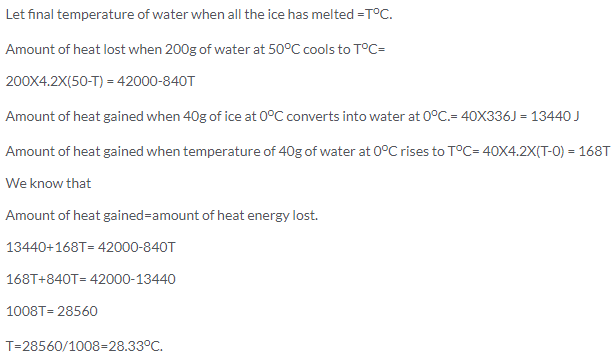Solution 8.Solution 9.

Since the whole block does not melt and only 2 kg of it melts, so the final temperature would be 0 oC.
Amount of heat energy gained by 2 kg of ice at 0oC to convert into water at 0oC=2 x 336000 = 672000 J
Let amount of water poured = m kg.
Initial temperature of water = 100oC.
Final temperature of water = 0oC.
Amount of heat energy lost by m kg of water at 100oC to reach temperature 0oC =m x 4200 x 100 = 420000m J
We know that heat energy gained = heat energy lost.
672000J = mX420000J
m = 672000/420000=1.6kg

Solution 10.

Amount of heat energy gained by 100 g of ice at -10C to raise its temperature to 0C = 100 × 2.1 × 10 = 2100 J
Amount of heat energy gained by 100 g of ice at 0C to convert into water at 0C = 100 × 336 = 33600 J
Amount of heat energy gained when temperature of 100 g of water at 0C rises to 100C = 100 × 4.2 × 100 = 42000 J
Total amount of heat energy gained is = 2100 + 33600 + 42000 = 77700 J = 7.77 × 104 J

Solution 11.

Amount of heat energy gained by 1kg of ice at -10oC to raise its temperature to 0oC= 1 x 2100 x 10 = 21000 J
Amount of heat energy gained by 1kg of ice at 0oC to convert into water at 0oC = L
Amount of heat energy gained when temperature of 1kg of water at 0oC rises to 100oC= 1 x 4200 x 100 = 420000 J
Total amount of heat energy gained = 21000 + 420000 + L = 441000 + L.
Given that total amount of heat gained is = 777000J.
So,
441000 + L = 777000.
L = 777000 – 441000.
L = 336000JKg-1Solution 12.

Mass of ice, mice = 200 g

Time for ice to melt, t1 = 1 min = 60 s

Mass of water, mw = 200 g

Temperature change of water, ΔT = 20 °C

Rate of heat exchange is constant. So, power required for converting ice to water is same as the power required to increase the temperature of water.

Exercise 11(C)

Solution 1.

The earth receives heat radiations from sun which reach us after passing through its atmosphere. The earth’s atmosphere is transparent for the visible and thermal radiations of short wavelengths coming from the sun. The earth’s surface and objects on it thus become warm in the day time. After the sunset, the earth’s surface and the objects on it radiate the infrared radiations of long wavelengths. A part of these radiations are reflected back by the clouds and a part of it is absorbed by the green house gases like carbon dioxide, methane, water vapours and chlorofluorocarbons. Thus the clouds and green house gases prevents a large fraction of radiations given out by the earth’s surface, from escaping into the space. This phenomenon is called greenhouse effect.

Solution 2.

Carbon dioxide, methane, water vapours and chlorofluorocarbons.

Solution 3.

Solution 4.

Coal, petroleum, natural gas.

Solution 5.

From sun, we receive 1366W m-2 energy at the top of our earth’s atmosphere, out of which only 235Wm-2 energy reaches near the earth’s surface. The earth and ocean surface absorbs 168 W m-2 energy and only 67 W m-2 energy remains in the lower atmosphere. With this much energy received on earth surface, its actual surface temperature would have been around -180C which is quite uncomfortable for human living. Fortunately the greenhouse gases present in the earth’s atmosphere contribute in trapping the heat energy within the atmosphere and they produce an average warming effect of about 330C to keep the effective temperature around 150C. So, greenhouse effect helps in keeping the temperature of earth’s surface suitable for living of human beings.

Solution 6.

Three reasons for increase of greenhouse gases:

1. The burning of fuels, deforestation and industrial production
2. Increase of population.
3. Imbalance of carbon dioxide cycle

Solution 7.

The effect of enhancement of greenhouse effect are:

1. The variable change in the climate in different parts of the world has created difficulty and forced the people and animals to migrate from one place to another place.
2. It has affected the blooming season of the different plants.
3. The climate changes have shown the immediate effect on simple organism and plants.
4. It has affected the world’s ecology.
5. It has increased the heat stroke deaths.

Solution 8.

Global warming means the increase in the average effective temperature of earth’s surface due to an increase in the amount of greenhouse gases in its atmosphere.

Solution 9.

The effect of enhancement of greenhouse effect are:

1. The variable change in the climate in different parts of the world has created difficulty and forced the people and animals to migrate from one place to another place.
2. It has affected the blooming season of the different plants.
3. The climate changes have shown the immediate effect on simple organism and plants.
4. It has affected the world’s ecology.
5. It has increased the heat stroke deaths.

Solution 10.

Due to rise in sea level the building and roads in the coastal areas will get flooded and they could suffer damage from hurricanes and tropical storms.

Solution 11.

Due to global warming, many new diseases have emerged because bacteria can survive better in increased temperature and they can multiply faster. It is extending the distribution of mosquitoes due to increase in humanity levels and their frequent growth in warmer atmosphere. This has resulted in increase of many new diseases. The deaths due to heat stroke have certainly increased.

Solution 12.

Due to global warming, many new diseases have emerged because bacteria can survive better in increased temperature and they can multiply faster. It is extending the distribution of mosquitoes due to increase in humanity levels and their frequent growth in warmer atmosphere. This has resulted in increase of many new diseases. The deaths due to heat stroke have certainly increased.

Solution 13.

At the present rate of increase of green house effect, it is expected that nearly 30% of the plant species will extinct by the year 2050 and up to 70% by the end of the year 2100. In the near future, warming of nearly 3oC will result in poor yield in farms in low latitude regions. This will increase the rise of malnutrition.

Solution 14.

At the present rate of increase in green house effect, is estimated that nearly 30% of the plant and animal species will extinct by the year 2050 and upto 70 % by the end of year 2100. This will disrupt ecosystem. The animals from the equatorial region will shift to higher latitude in search of cold regions. The absorption of carbon dioxide by ocean will cause acidification due to which marine species will migrate.

Solution 16.

The tax calculated on the basis of: carbon emission from industry, number of employee hour and turnover of the factory is called carbon tax.
This tax shall be paid by industries. This will encourage the industries to use the energy efficient techniques.

Solution 1 (MCQ).

-18oC

Solution 2 (MCQ).

The increase in sea levels.
Explanation: Due to global warming, the average temperature of the Earth has increased and has lead to the melting of ice around both the poles. This melting of ice has lead to an increase in the level of water in sea.

More Resources for Selina Concise Class 10 ICSE Solutions